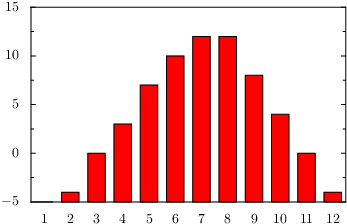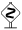# PyX — Example: bargraphs/minimal.py

0.2 KB
36.5 KB
0.2 KB
38.5 KB
18.5 KB
23.9 KB

## Minimal bar-graph example```from pyx import *

g = graph.graphxy(width=8, x=graph.axis.bar())
g.plot(graph.data.file("minimal.dat", xname=0, y=2), [graph.style.bar()])
g.writeEPSfile("minimal")
g.writePDFfile("minimal")
g.writeSVGfile("minimal")
```

### Description

For a minimal bar plot you have set an bar axis in the graph constructor and provide Xname column data (X stands for the bar axis to be used). Here, we just use column 0 which is automatically filled by `graph.data.file` with the line number of the corresponding entry. Furthermore, you need to specify the graph style, since the default graph styles `symbol` and `function` (depending on the data type) are not appropriate for bar graphs.

Note that bar graphs differ from other xy-graphs in that they use discrete axes for one graph dimension. However, the only affected components of this fundamental change are one of the axes, which needs to be come a discrete one, i.e. a bar axis, and the usage of appropriate graph styles.A bar graph is fundamentally different from a graph with a histogram style in its usage of a discrete axis in one graph dimension. A histogram instead is created using continuous axes in all graph dimensions and just drawing the data in a specific bar-graph-like presentation. In particular, the discreteness of the bar axis is reflected in the naming of its column name: instead of the continuous "X" it expects an "Xname" (where X stands for the bar axis used) as mentioned above.As all axes and graph dimensions in the PyX graph system are treated equally all you need to modify to get bar graph with horizontal bars is to assign the bar axis to the y-axis in the graph constructor and change the names of the data columns to `yname` and `x`.By using the bar style you implicitly also select a different positioning style, namepy `barpos`. This positioning style handles a single-nested axes with sub-axis values of the range going from 0 to 1.